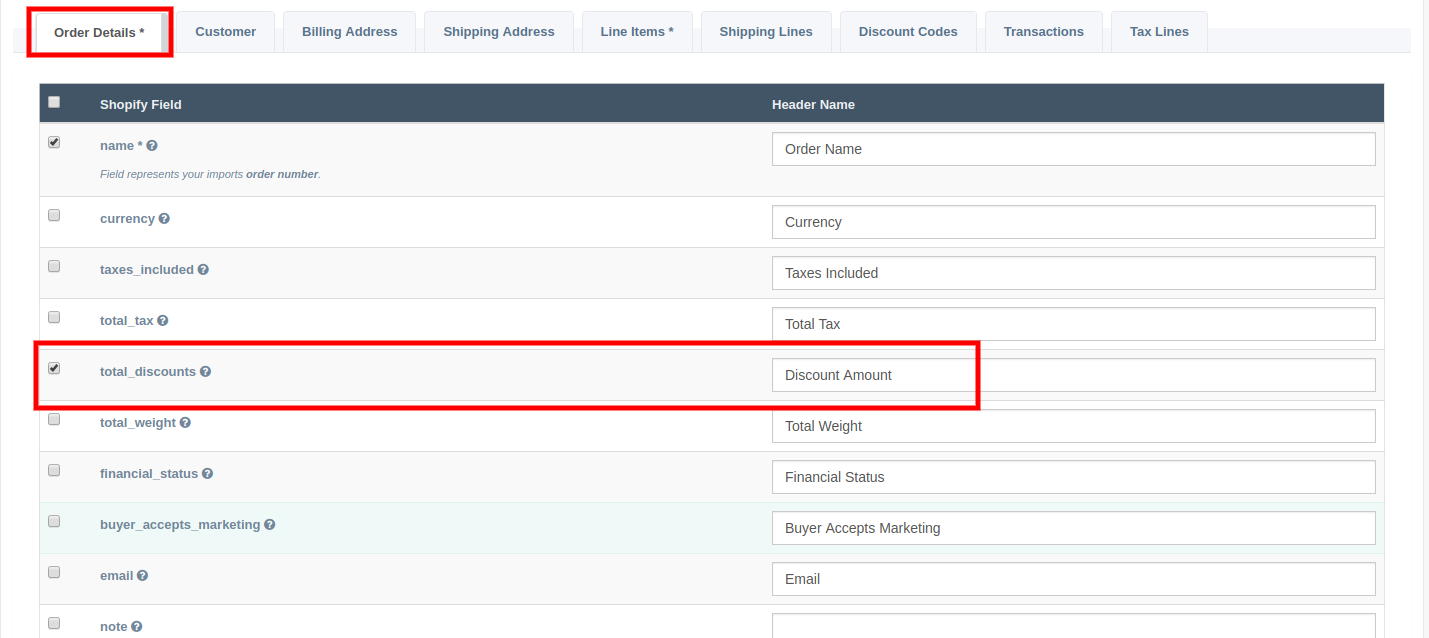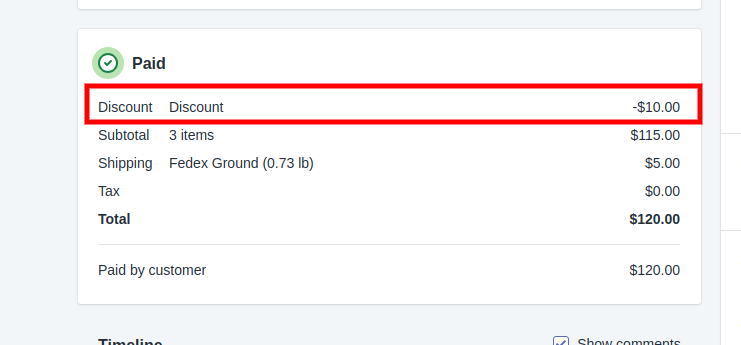## How do I import my Discount Codes?

When adding discounts it's important to understand how the discount will display on your order. There are two areas to add discounts when importing an order.

### Total Discounts

The total_discounts field on the "Order Details" tab of the data mapping is used to apply the total discount to your order. Mapping this column to Shopify will apply the discount to the Order. Even though the discount is now applied to your order, you will NOT see the discount on the Shopify order admin. The total_discounts field only tells Shopify to apply the discount to the order.In order to see the discount lines on your order you must also fill in the discount_codes.amount. You can also fill out the discount_codes.code if you want to add a label for the discount code. To add the type of discount to your order you can import the discount_code.type. For example, if you want to apply a discount code as a percentage than you need to import percentage as the value. The default value is fixed_amount which will apply the amount to your order.

Once these fields are filled in you will see the line item for the discounts that you've applied to your order. The discount codes show up in the financial section of the Shopify order admin. This is the section right below the list of your line items.As you can see in our example, we applied a discount of \$10.00 US Dollars to our order which was originally \$125.00 US Dollars.

### Total Discount Format

One area of confusion when applying a total_discount is the format of the value you need to provide. When sending the total_discount or discounts.amount field you must provide a positive integer value or you'll get an error from Shopify. Do not provide a negative number (e.g. -5.00) or a number wrapped in parenthesis (e.g. (5.00)). The format must be a positive decimal value (e.g. 5.00). If you provide a value that is not a decimal or is negative you'll get one of the following errors from Shopify:

1. expected String to be a float
2. 'discount_codes Amount must be greater than or equal to 0', 'Total discounts must be greater than or equal to 0

NOTE: At this time Shopify only supports applying a single discount_code to an order.

App: EZ Importer

Tags: faq, orders, discounts